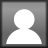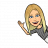March 15

Why did two 4s skip lunch? They already 8!

Who doesn’t love a good math pun?

And it’s perfect timing for our multiplication and division unit! As we embark on this next unit, it’s extremely important that students are practicing their multiplication facts daily, or as close to that as possible. As a guide, we will be focusing on specific facts each week, however that doesn’t mean a student couldn’t spend more time on a fact, or move on if they’ve mastered it. It’s all about finding out which numbers need extra work, and persevering till you’ve mastered it! We will be practicing all our multiplication facts up to 12×12.

Here are different things you can do to practice the facts:

1. Make Flashcards. This is much better than buying them premade! Writing the facts down is in itself a form of practice. Students can cut the cards, write the multiplication sentence on one side, and the answer on the back.
2. Math rap songs. For our auditory learners, listening to songs about multiplication and adding a beat to it can be helpful. Students can learn all the newest pop songs by heart…why not their multiplication facts? YouTube has tons of options. You just need to find the one that appeals to you.
3. Online multiplication practice. These are a few suggestions but there are TONS to choose from.
4. Printed practice sheets. I have heaps of practice sheets that students can take home.
5. Find tricks to help you remember your facts. I will share tricks each week as well.

This coming week we will be focusing on the easier facts to get them out of the way!

O, 1, 2, 5, 10

Tips and Tricks:

0 – Every number multiplied by zero is zero!   Ex:    0 x 1 = 0      0 x 12 = 0     7 x 0 = 0

1 – Every number multiplied by 1 stays the same!  Ex:   1 x 3 = 3    7 x 1 = 7    11 x 1 = 11

2 – Add the number to itself…in other words, double it!  Ex:   2 x 4 = 8 because 4 + 4 = 8

5 – There are a few different tricks for 5.

i) Cut the number in half, then multiply by 10. Ex:  5 x 6,   half of 6 is 3,  3 x 10 = 30  therefore, 5 x 6 = 30

ii) Multiply the number by 10, then cut in half.  Ex: 5 x 3,   10 x 3 = 30,  half of 30 is 15, therefore 5 x 3 = 15

iii) The last digit follows the pattern 5, 0, 5, 0… Ex: 5, 10, 15, 20…

iv) Think of a clock. The numbers on the clock represent the minutes in increments of 5. When the minute hand is on the 4, it’s 20 minutes. Therefore, 5 x 4 = 20

10 – Add a zero to the end.  Ex:   3 x 10 = 30     6 x 10 = 60    12 x 10 = 120

Tags:

Posted March 15, 2019 by melissathompson in category Homework, Math

3 thoughts on “Why did two 4s skip lunch? They already 8!”

1.Miriam Friedman

Thanks! Yaakov loves puns! He makes great ones too!

2.griffin

this homework is quite a number! (see what I did there?

1.melissathompson (Post author)

You’re always good for a pun, Griffin!